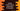# Python tutorial : Part 5 : python math modulePython Math module:

Using Math module in python, we can access to different mathematical functions already defined by the C standard . “math” module functions cannot use with complex numbers. For complex numbers, another module known as “cmath” (link) is available.

To use this module , we need to include “import math” for that program. e.g. :

``````import math
print math.pi``````

Following are the functions provided by math module :

``````ceil(x) ,return smallest integer value greater than or equal to x

copysign(x,y) , returns x with the sign of y . e.g. copysign(1.0, -2.0) returns -1.0

fabs(x) : returns absolute value of x

factorial(x) : returns x factorial .If x is not integral or negative, it throws an error.

floor(x) : returns the largest integer value less than or equal to x

fmod(x,y) : returns reminder when x is divided by y

frexp(x) : Return the mantissa and exponent of x as the pair (m, e). m is a float and e is an integer such that x == m * 2**e

fsum(iterable) : Return an accurate floating point sum of values in the iterable.

isinf(x) : Checks if the float x is positive or negative infinity.

isnan(x) : checks if the float x is NaN ( Not a number )

ldexp(x,i) : return x * (2**i). This is essentially the inverse of function frexp().

modf(x) : returns the factional and integer parts of x.

trunc(x) : returns the truncated int value of x``````

Power and logerithmic functions :

``````exp(x) : returns e**x

expm1(x) : returns e**x - 1

log(x[, base]) : returns the logarithm of x to the base ( default base is e )

log1p(x) : return the natural logarithm of 1+x (base e).

log10(x) : return the base-10 logarithm of x

pow(x,y) : return x raised to the power y

sqrt(x) : return the square root of x``````

Trigonometric functions :

``````acos(x) : return the arc cosine of x in radians

asin(x) : return the arc sine of x in radians

atan(x) : return the arc tangent of x in radians

atan2(y,x) : Returns atan(y / x)

cos(x) : returns the cosine of x

hypot(x, y) : Return the Euclidean norm, sqrt(x*x + y*y).

sin(x) : return the sine of x radians

tan(x) : return the tangent of x radians``````

Angular Conversion :

``````degrees(x) : Converts angle x from radians to degrees

radians(x) : Converts angle x from degrees to radians``````

Hyperbolic functions:

``````acosh(x) : Returns the inverse hyperbolic cosine of x

asinh(x) : Returns the inverse hyperbolic sine of x

atanh(x) : Returns the inverse hyperbolic tangent of x

cosh(x) : Returns the hyperbolic cosine of x

sinh(x) : Returns the hyperbolic cosine of x

tanh(x) : Returns the hyperbolic tangent of x``````

Special Functions :

``````erf(x) : Returns the error function at x

erfc(x) : Returns the complementary error function at x

gamma(x) : Returns the Gamma function at x

lgamma(x) : Returns the natural logarithm of the absolute value of the Gamma function at x``````

Constants :

``````pi: Mathematical constant to available precision (3.14159...)

e : mathematical constant e to available precision (2.71828...)``````